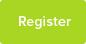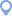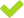# Alin (Student in London)

Alin
StudentLondon, London
Member Since: 11/02/2016
Home Town: LondonTag this StudentReport Inappropriate Content

 Primary KS3 GCSE A-Level Degree Maths - - - -Hi I am a student studying degree level maths.

My maths was intensely trained in China but I am looking for improvement to boost my future career path.

1. Show for any positive real number a,b,c, We have :
1<a/Sqrt(a^2+b^2)+b/Sqrt(b^2+c^2)+c/Sqrt(c^2+a^2)

3/Sqrt(2)>a/Sqrt(a^2+b^2)+b/Sqrt(b^2+c^2)+c/Sqrt(c^2+a^2)

2. Find the first derivative of f(x)= x^x

Thank you.

Availability: Weekends

Willing to travel: 5 miles